# Refinement of Token Distribution Math

in #fractally14 days ago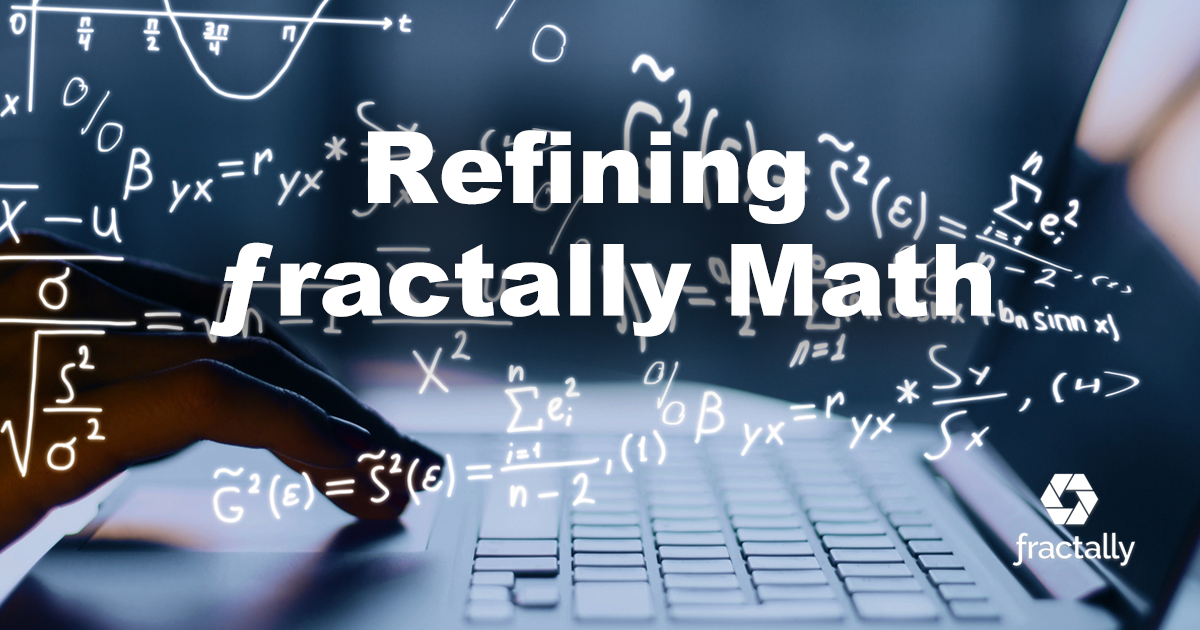This post is a follow-up to my post Fractally White Paper Addendum 1, where the math described is more thoroughly modeled. It is designed to honor the intent of the original post, and the proposal approved by the Genesis Fractal.

# Supply Curve

The issuance rate math was refined to represent a continuous radio-active decay function with a 1-year half-life utilizing the equation: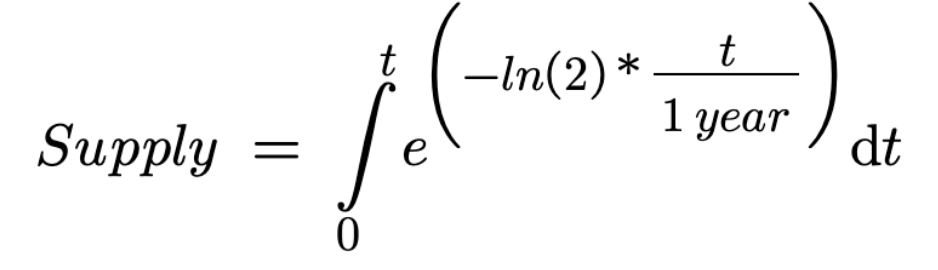This allows us to calculate the exact supply on a microsecond to microsecond basis or whatever resolution is desired. The resulting curve looks like this: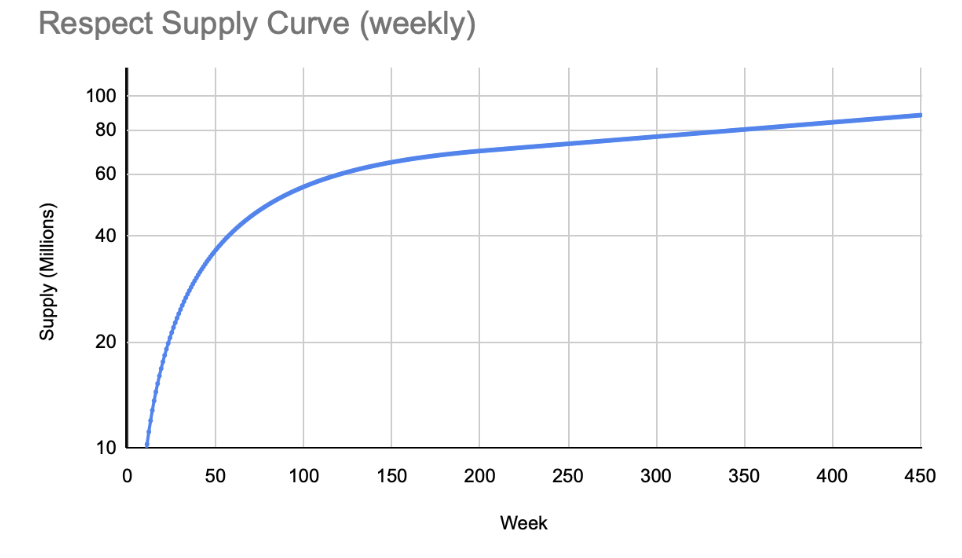The emission curve changes once the instantinous APR falls to 5% which occurs after about 4 years.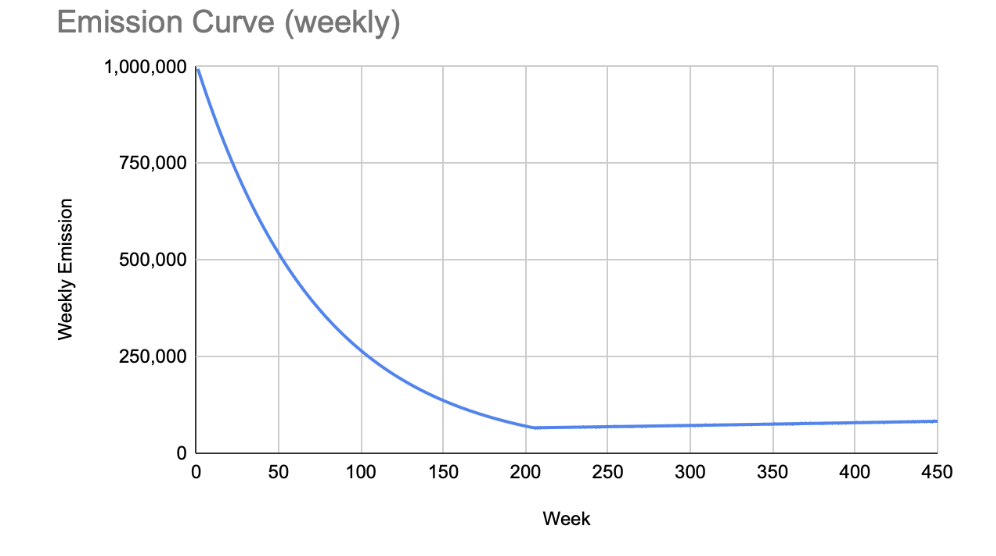For the purpose of migrating the genesis fractal to this new curve we will take the total amount of respect defined by the curve for 22 weeks and divide it on a pro-rata basis according to the amount of respect earned under the old method. This will result in everyone having the same percentage of the respect at the start.

# Weekly Respect Allocation

Now that we have defined what the global supply of respect will be at any given moment in time we can discuss the algorithm used to distribute the new respect. The prior post called for replacing fibonacci with this function: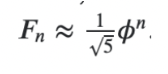But that function did not produce the result of level 0 earning 0 respect. The equation was tweaked to intersect with 0.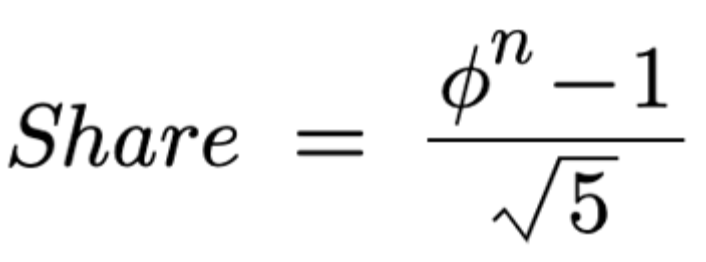Some people have asked about whether or not we should still start the Fibonacci sequence at position 2. Now that we are using an approximation to Fibonacci there is no longer a need to do this. The relative share of the inflation each person earns is the same regardless of where we shift the function along the x axis.

## Initial Average

The new algorithm requires an initial average in order to calculate a new average. We will initialize everyone's average according to the sum of the level earned in the past 6 weeks divided by 6. This average will then be updated according to formula ` avgnew = (5 * avgold + level)/6` going forward which matches the following curve from the original proposal.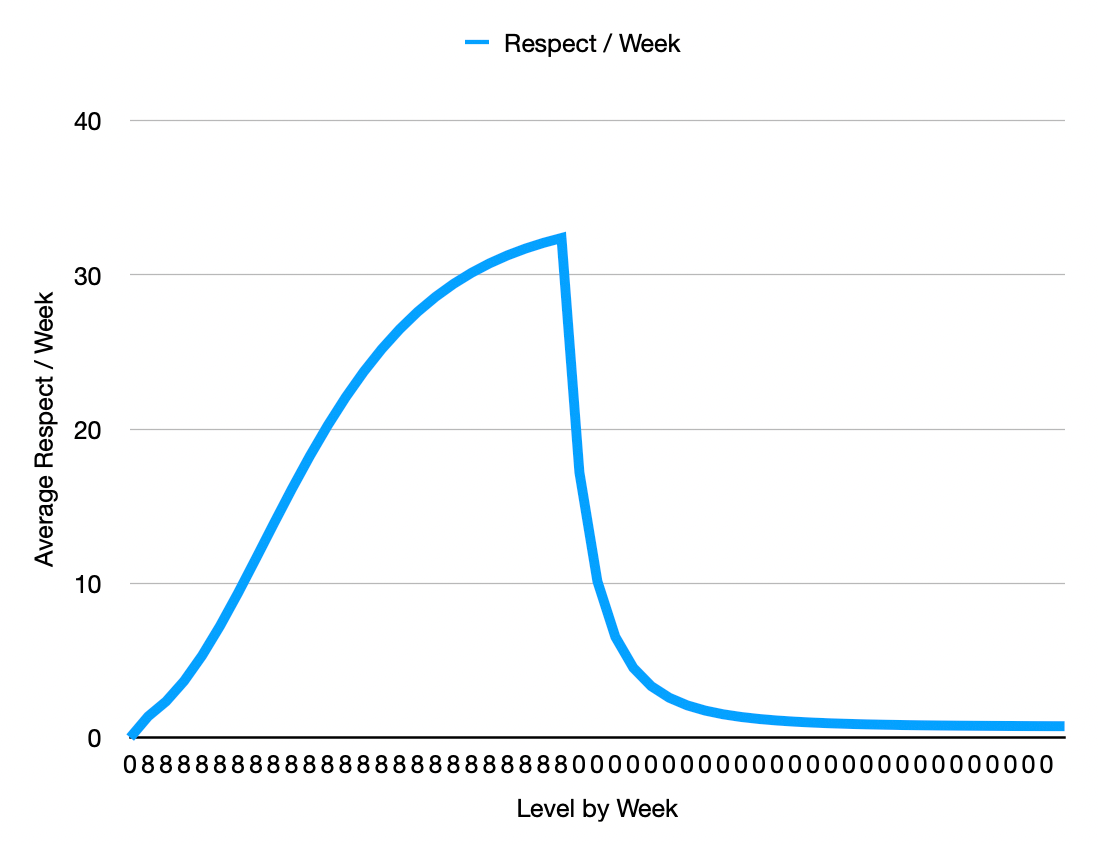## Other Uses of Inflation

The ƒractally white paper calls for inflation to be utilized to fund many different things including: weekly participation, teams, staking, social media contributions, and liquidity rewards. Regardless of how many different things are funded by inflation, the total supply of respect will follow the supply curve defined at the start of this article.

The numbers derived from `fib( avg(level) )` represents "shares in the inflation" and not the actual amount of respect earned. Any member who is on a team will see their team get a matching amount of "shares in the inflation". Only after totalling all "shares in the inflation" can we calculate each persons pro-rata share of the new respect.

For clarity, every member receives respect every week whether or not they participate, and they cease to be a member if they haven't participated in 12 consecutive weeks. They start at 0 for their initial average if they are invited back. Their team also earning matching shares in the inflation. So to understand how much respect will be earned requires factoring in the team share. If all members were on a team this would be 50%.

In a subsequent week we will propose a change in how many inflation shares are allocated to each team. With this new mathematical model that change will have no impact on the relative percent of inflation being earned by members in the weekly meeting.

There will likely be additional clarifications in future weeks as we reduce the ideas to deterministic smart contracts. As always, the community has the power to reach consensus on whether these changes honor the intent of the existing consensus and, if necessary, can propose and ratify an alternative.

перевод на русский язык - https://hive.blog/fractally/@shakhruz/utochnenie-matematiki-raspredeleniya-tokenov.

Подписывайтесь на мой телеграм канал чтобы узнавать подробнее о Фракталли и многом другом на русском языке.

rePOSTed

I repost things like this on my timeline... from time to time.
Regards 🥓